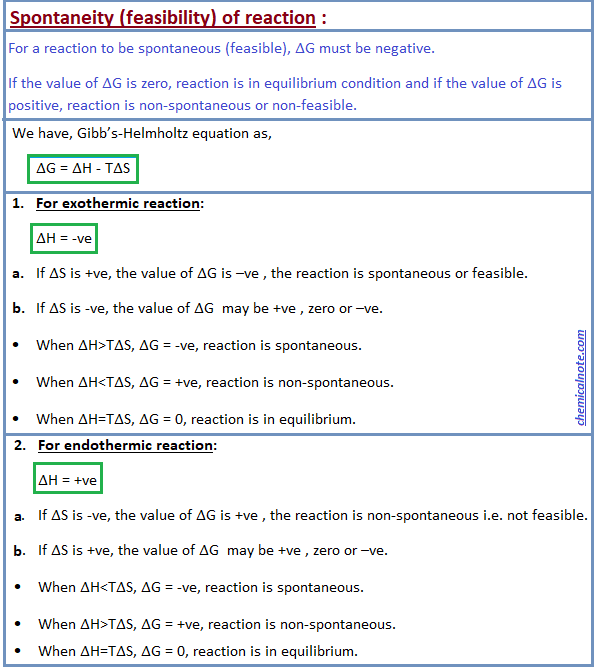• Post Author:

#### What is chemical thermodynamics?

Thermodynamics is the branch of physical science that deals with the relationships between heat and other forms of energy (such as mechanical, electrical or chemical energy).

In broad terms, thermodynamics deals with the transfer of energy from one place to another and from one form to another.

#### Spontaneous and non-spontaneous process

Spontaneous process:

A process which may take place by itself or by initiation is called a spontaneous process.

In other words, a process which can take place by itself or has a tendency to take place is called spontaneous process.

Spontaneous process is simply a process which is feasible.

Examples of spontaneous process :

• Dissolution of common salt in water.
• Flow of water down a hill.
• Flow of heat from hot body to a cold body.
• Combination of H2 and I2 to form HI.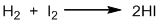• Combination of hydrogen and oxygen to form water (when initiated by passing an electric spark).
• Reaction between CH4 and O2 to form CO2 and H2O (when initiated by ignition).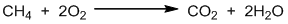Non-spontaneous process:

A process which can neither take place by itself nor by initiation is called a non-spontaneous process.

Examples of non-spontaneous process:

• Dissolution of sand in water.
• Flow of water up a hill.
• Flow of heat from low pressure to a high pressure.

#### Entropy

Entropy is a thermodynamic state quantity that is a measure of the randomness or disorderness of the system. It is denoted by the letter ‘S’.

Greater the disorderness in molecules, greater will be the entropy. Generally, entropy is inversely proportional to the intermolecular force of attraction. Hence,

∆Sgas > ∆Sliq > ∆Ssolid

Mathematically,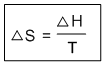Standard entropy: Entropy at standard condition i.e. 250C, 1 atm pressure and 1 molar concentration is called standard entropy. It is denoted by ∆S0.

Types of entropy:

1. Entropy of vaporization: The entropy change when one mole of a liquid changes into vapour (gas) is called entropy of vaporization.

2. Entropy of fusion: The entropy change when one mole of a solid changes into liquid is called entropy of fusion.

3. Entropy of sublimation: The entropy change when one mole of a solid changes into vapour (gas) is called entropy of sublimation.

4. Entropy of combustion or oxidation: The entropy change when one mole of any substance is completely burnt or oxidized is called entropy of combustion or oxidation.

Limitations of first law of thermodynamics:

> This law only explain about the total heat content in the system but does not say anything about the direction of flow of heat.

> This law does not say whether the process (reaction) is spontaneous or not.

#### Second law of thermodynamics

Second law of thermodynamics can be defined in a number of ways as follows:

Kelvin Plank statement: It is impossible to construct an engine operating in a complete cycle which will convert all the heat energy into work i.e. no any system (or engine) will have 100% efficiency.

Clausius statement: It is impossible for a self acting machine to transfer heat from a body at lower temperature to a higher temperature without any external actions (agencies).

In terms of entropy: “In any spontaneous process, there is always an increase in entropy of the universe”.

In other words- “It is not possible to have a process in which the entropy of an isolated system is decreased”.

#### Entropy and spontaneity

According to second law of thermodynamics, a process will occur spontaneously if entropy of the universe increases. Therefore;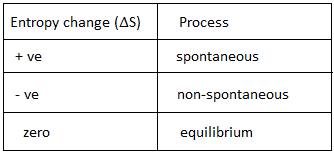#### Gibb’s free energy

Amount of energy available( required) to perform a certain work is called free energy. Generally, the amount of heat energy required to perform a certain work is called Gibb’s free energy. It is denoted by letter ‘G’.

Mathematically,

G = H – TS

Where,

H= Enthalpy(heat content)

S= Entropy of the system

T = The absolute temperature.

#### Gibb’s- Helmholtz equation

According to definition of Gibb’s free energy,

G = H – TS —(i)

Let us consider G1, H1 and S1 be the initial and G2, H2 and S2 be the final free energy, enthalpy and entropy respectively. Then, for initial state:

G1 = H1 – TS1 —(ii)

Similarly, for final state:

G2 = H2 – TS2 —(iii)

From equation (ii) and (iii),

G2 – G1 = (H2-TS2) – (H1-TS1)

Or, G2 – G1 = H2-TS2 – H1 + TS1

Or, G2 – G1 = (H2– H1) – T(S2 – S1)

Or, ∆G = ∆H – T∆S —-(iv)

This equation (iv) is called Gibb’s-Helmholtz equation.

#### Standard free energy

Free energy change in between reactant and product at standard conditions i.e. 250C, 1 atm pressure and 1M concentration is called standard free energy change. It is denoted by ∆G0.

Mathematically,

∆G0 = ∆H0 – T∆S0

For chemical reaction,

∆G0 = Ʃ∆G0product – Ʃ∆G0reactant

Free energy is also defined as thermodynamic function of a system which indicates the capacity of maximum useful work that can be done by the system.

#### Spontaneity (feasibility) of reaction on the basis of Enthalpy, Entropy and Gibb’s free energy

A reaction which occurs itself is called spontaneous or feasible reaction.